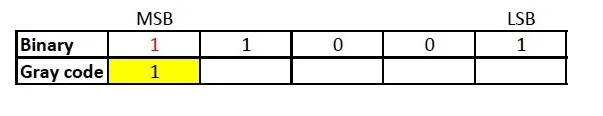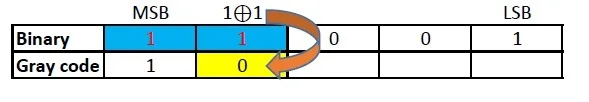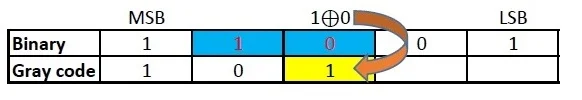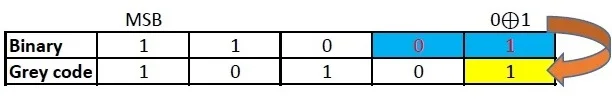# How to convert octal number to gray code

## Octal Number

Octal numbers use digits from 0-7 only. It is known as base-8 number. The place value of each digits of an octal number varies as the whole number powers of 8 starting from the right (Least Significant Digit). The first single digit number in octal system is 0 and the last is 7. Similarly, the first two digit octal number is 10 and the last is 77 and so on. Octal number system was widely used in early computers.

## Gray code

Gray code, also known as reflected binary code, is a code having digits 0 and 1. Gray code do not have place value for its digits. Any successive codes in Gray code system have only one bit changes.

## How to convert octal to gray code

Octal number cannot be directly converted to Gray code. Hence, to convert octal to gray code, first, convert the octal number to binary. Then, convert the binary to gray code.

Octal number to gray conversion can be well understood from the following example:

### Convert 318 to gray code

#### Step 1: To Convert 318 to gray code at first convert 318 to binary.

To convert octal to binary write down the binary code corresponding to each digit of octal number.

 Octal 3 1 Decimal 11 001

Hence, 318 = 110012

### Read: How to convert Octal number to Binary

#### Step 2: Now, Convert 110012 to gray code.

The MSB (Most Significant Bit) of a gray code and binary code will be the same.The next digit of gray code will be the EXOR of the MSB and the digit right to the MSB of the binary code.Similarly EXOR the digit in place and the previous digit of binary code to obtain the next digit of gray codeRepeat the previous step.Repeat the previous step till the LSB of gray code is found.Therefore, Gray code corresponding to 318 = 10101